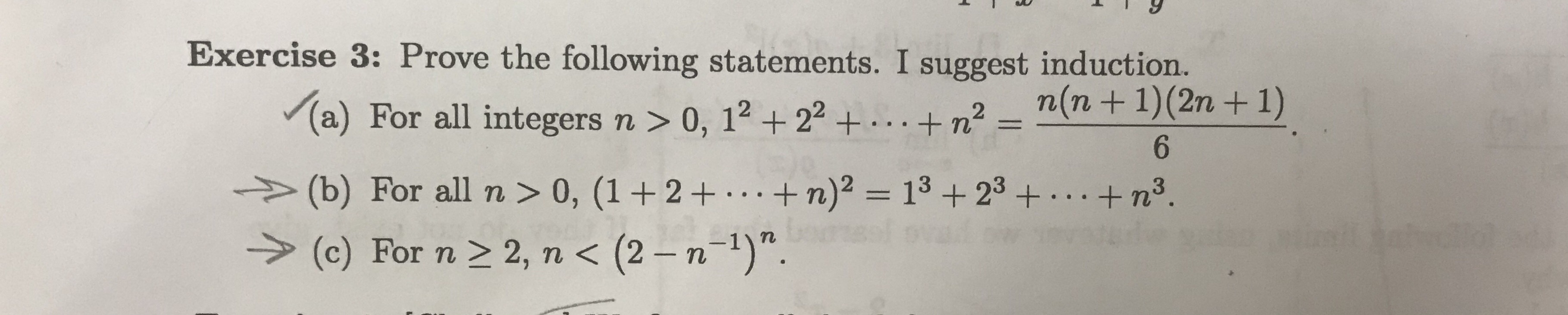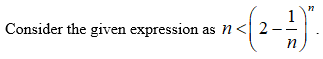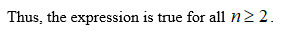# Exercise 3: Prove the following statements. I suggest induction.n(n+1)(2n+ 1)(a) For all integers n > 0, 12+ 22 ++n26(b) For all n > 0, (1 +2 +...+ n)2 = 13 +23n3.(c) For n 2 2, n < (2 - n-1)".

Question

Induction proof for part chelp_outlineImage TranscriptioncloseExercise 3: Prove the following statements. I suggest induction. n(n+1)(2n+ 1) (a) For all integers n > 0, 12+ 22 ++n2 6 (b) For all n > 0, (1 +2 +...+ n)2 = 13 +23 n3. (c) For n 2 2, n < (2 - n-1)". fullscreen
check_circleExpert Solution
Step 1Step 2

First prove that the given expression is true for n = 2.

Step 3Now assume that the given expression is true for all n = k.

...

### Want to see the full answer?

See Solution

#### Want to see this answer and more?

Solutions are written by subject experts who are available 24/7. Questions are typically answered within 1 hour*

See Solution
*Response times may vary by subject and question
Tagged in

### Other